# The Math Behind the Beauty

By M. BourneJessica Simpson

What has mathematics got to do with beauty? Actually, a lot. Physical attraction depends on ratio, and in particular symmetry. (See Facial attractiveness: evolutionary based research.)

Our attraction to another person's body increases if that body is symmetrical and in proportion. Likewise, if a face is in proportion, we are more likely to notice it and find it beautiful.

Scientists believe that we perceive proportional bodies to be more healthy. This is suggested in the following famous drawing by da Vinci showing an idealized human body within a square and a circle.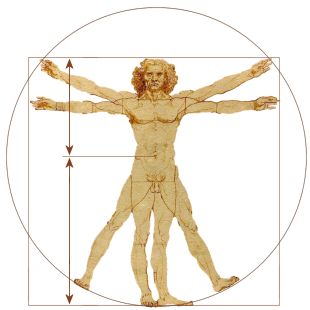Leonardo da Vinci's "Vitruvian Man",
showing the navel proportion

Leonardo da Vinci's drawings of the human body emphasised its proportion. Many have claimed the ratio of the following distances in the above Vitruvian Man image is the Golden Ratio:

(foot to navel) : (navel to head) ≈ 1.618:1

However, it's not that close at 1.505:1.

Similarly, it is believed buildings may be more attractive to some people if the proportions used follow the Golden Ratio.

Continues below

## Golden Ratio

The Golden Ratio (or "Golden Section") is based on Fibonacci Numbers, where every number in the sequence (after the second) is the sum of the previous 2 numbers:

1, 1, 2, 3, 5, 8, 13, 21, ...

We will see (below) how the Fibonnaci Numbers lead to the Golden Ratio:

Φ = 1.618 033 ...

## Physical Beauty

Why do many people feel that Jessica Simpson is beautiful?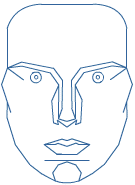Image source

This mask of the human face is based on the Golden Ratio. The proportions of the length of the nose, the position of the eyes and the length of the chin, are claimed to conform to some aspect of the Golden Ratio.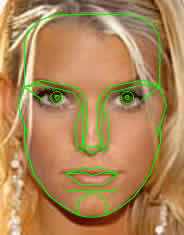When placed over the photo of Jessica Simpson, we see there is a good fit (that is, the proportions of her face fit the geometrically "nice" proportions of the mask, based on the Golden Ratio).

Her beauty is mathematical!

## The Fibonacci Sequence

Let's look at the ratio of each number in the Fibonacci sequence to the one before it:

1/1 = 1

2/1 = 2

3/2 = 1.5

5/3 = 1.666...

8/5 = 1.6

13/8 = 1.625

21/13 = 1.61538...

34/21 = 1.61905...

55/34 = 1.61764...

89/55 = 1.61861...

If we keep going, we produce an interesting number which mathematicians call "phi" (Golden Ratio or Golden Section):

Phi = 1.618 033 988 7...

This ratio was used by architects and artists throughout history to produce objects of great beauty (like Michelangelo's "David" and the Greek temples.)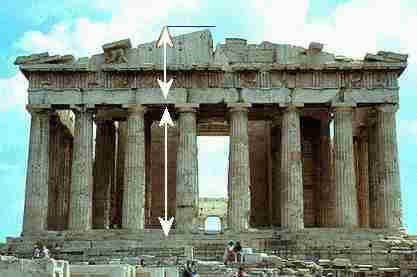The Parthenon in Greece. The ratio of the distances indicated is the Golden Ratio.

Note: The choice of the arrow placement in the above image is somewhat arbitrary. The bottom of the lower arrow is placed one one of the steps leading up to the main building, which is not overly convincing.

Phi (Φ) is like pi (π) in the sense that it is an irrational number. There is no equivalent fraction for Φ and its decimal keeps going and never stops.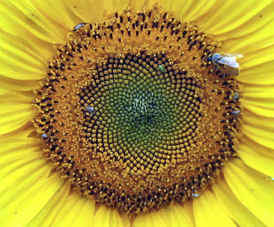Sunflower (Image source)

The Golden Ratio also occurs in nature, in the patterns we sometimes see in sunflowers, pine cones and so on. This is largely because one of the best ways to efficiently pack things tightly together is using the Fibonacci sequence.

### Conclusions

The conection with physical attractiveness and the Fibonacci sequence is interesting to consider, but is not always conclusive, and not particularly scientifically valid. See: Is Phi a Fibonacci furphy?

In many cases, it's probably a matter of finding the pattern you are looking for, rather than a meaningful observation. In short, it's a bit of fun, and not to be taken too seriously.

Search IntMath

### Online Algebra Solver

This algebra solver can solve a wide range of math problems.

### Algebra Lessons on DVD

Math videos by MathTutorDVD.com

Easy to understand algebra lessons on DVD. See samples before you commit.